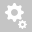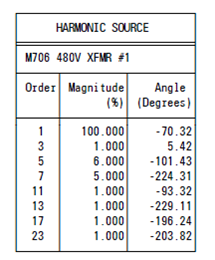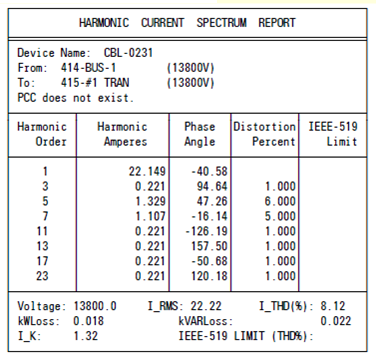##Recently viewed tickets• Sales
• Library Model Request
• Technical Support

# Calculate Harmonics Current Angle

Here is the equation PTW32 used for harmonics current angle:

Ang = HiwaveAngleintheLib + order*(LFangle4FirstOrder - FirstOrderAngleintheLib)

For the 3rd harmonics in the example below:

Ang3 = 5.42 + 3*(-40.58 – (-70.32)) = 94.64